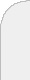inicioir al DIIbuscaran introduction to stochastic integrals When including white noise into the model a simple evolution equation becomes intractable for the classic theory of ODE's. We cannot describe the dynamics using a deterministic solution anymore, and stochastic processes and probability theory have to be used. The stochastic process that naturally arises in this setting is the so called Ito integrals. In this talk we will motivate the definition of Ito integrals,we will present its rules of calculus and we will underline itsconnection to second order partial differential equations. This last point closes the circle. It allows us to use powerful tools of PDE's to solve stochastic differential equations, andit allows us to use the robust martingale theory to study some second order PDE's.